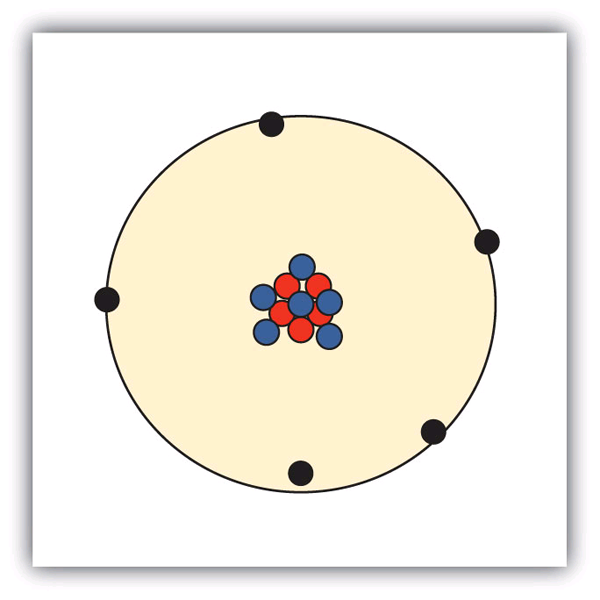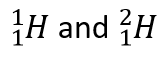# Basic ATOMIC THEORY Exercises

## Basic ATOMIC THEORY Exercises

Basic ATOMIC THEORY Exercises

List the three statements that make up the modern atomic theory.

Explain how atoms are composed.

Which is larger, a proton or an electron?

Which is larger, a neutron or an electron?

What are the charges for each of the three subatomic particles?

Where is most of the mass of an atom located?

Sketch a diagram of a boron atom, which has five protons and six neutrons in its nucleus.

Sketch a diagram of a helium atom, which has two protons and two neutrons in its nucleus.

Define atomic number. What is the atomic number for a boron atom?

What is the atomic number of helium?

Define isotope and give an example.

What is the difference between deuterium and tritium?

Which pair represents isotopes?

b)  26F and 25M

c)  14S and 15P

14.  Which pair represents isotopes?

a)  20C and 19K

b)  26F and 26F

c)  92U and 92U

15.  Give complete symbols of each atom, including the atomic number and the mass number.

a)  an oxygen atom with 8 protons and 8 neutrons

b)  a potassium atom with 19 protons and 20 neutrons

c)  a lithium atom with 3 protons and 4 neutrons

16.  Give complete symbols of each atom, including the atomic number and the mass number.
a)  a magnesium atom with 12 protons and 12 neutrons

b)  a magnesium atom with 12 protons and 13 neutrons

c)  a xenon atom with 54 protons and 77 neutrons

17.    Americium-241 is an isotope used in smoke detectors. What is the complete symbol for this isotope?

18.    Carbon-14 is an isotope used to perform radioactive dating tests on previously living material. What is the complete symbol for this isotope?

19.  Give atomic symbols for each element.

a)  sodium

b)  argon

c)  nitrogen

20.  Give atomic symbols for each element.

a)  silver

b)  gold

c)  mercury

d)  iodine

21.  Give the name of the element.

a)  Si

b)  Mn

c)  Fe

d)  Cr

22.  Give the name of the element.

a)  F

b)  Cl

c)  Br

d)  I

1.

All matter is composed of atoms; atoms of the same element are the same, and atoms of different elements are different; atoms combine in whole-number ratios to form compounds.

3.

A proton is larger than an electron.

5.

proton: 1+; electron: 1−; neutron: 0

7.9.

The atomic number is the number of protons in a nucleus. Boron has an atomic number of five.

11.

Isotopes are atoms of the same element but with different numbers of neutrons.are examples.

13.

a)  isotopes

b)  not isotopes

c)  not isotopes

15.

b)  19K

17.

95A

19.

a)  Na

b)  Ar

c)  N

d)  Rn

21.

a)  silicon

b)  manganese

c)  iron

d)  chromium